# CBSE Physics Practicals for Class 12

CBSE Physics Practicals for Class 12 play an important role in the assessment, adding to the final marks of the subject. As the board exam approaches, students go through the syllabus prescribed by the CBSE board. Besides this, they should also focus on the CBSE practicals for Class 12 syllabus carrying 30 marks. Preparing for the practicals will help them score better marks in Physics. For that, students must know to perform all the experiments given in the syllabus in order to understand all the concepts of CBSE 12th standard Physics in a detailed way. Students can also download the Physics Practical Class 12 Syllabus PDF for free here.

Physics Practical for Class 12 is divided into two sections, with marks distributed based on the experiment. In section A, six experiments are present in the practical exam. The experiment records and activities consist of 2 marks, and the viva on the experiment consists of 5 marks. Here, students will find all the experiments and activities to be performed in sections A and section B. Also, we have provided the Physics Lab Manual of CBSE Class 12, which consists detailed explanation of each experiment. To download the CBSE Syllabus for Class 12 Physics Practicals, click on the link below.

## CBSE Syllabus for Class 12 Physics Practical: Evaluation Scheme

Below you will find the CBSE Class 12 Physics Practical syllabus for students.

 Topic Marks Two experiments, one from each section 7 + 7 Practical record (experiment and activities) 5 One activity from any section 5 Investigatory Project 3 Viva on experiments, activities and project 5 Total 30

## CBSE Class 12 Physics Practical Syllabus: Experiments

Physics Practicals for Class 12 CBSE are given here so that students can understand the experiments in a better and more detailed way. Students are suggested to study the theory and law behind the experiment properly from Physics Lab Manual Class 12 before performing the experiment.

### CBSE Class 12 Physics Experiments

Section A
1. To determine the resistivity of two / three wires by plotting a graph for potential difference versus current.
2. To find the resistance of a given wire / standard resistor using a metre bridge.
3. To verify the laws of combination (series) of resistances using a metre bridge.
OR
To verify the laws of combination (parallel) of resistances using a metre bridge.
4. To determine the resistance of a galvanometer by the half-deflection method and to find its figure of merit.
5. To convert the given galvanometer (of known resistance and figure of merit) into a voltmeter of desired range and to verify the same.
OR
To convert the given galvanometer (of known resistance and figure of merit) into an ammeter of desired range and to verify the same.
6. To find the frequency of AC mains with a sonometer
Section B
1. To find the value of v for different values of u in the case of a concave mirror and to find the focal length.
2. To find the focal length of a convex mirror using a convex lens.
3. To find the focal length of a convex lens by plotting graphs between u and v or between 1/u and 1/v.
4. To find the focal length of a concave lens using a convex lens.
5. To determine the angle of minimum deviation for a given prism by plotting a graph between the angle of incidence and the angle of deviation.
6. To determine the refractive index of a glass slab using a travelling microscope.
7. To find the refractive index of a liquid using a convex lens and plane mirror.
8. To find the refractive index of a liquid using a concave mirror and a plane mirror.
9. To draw the I-V characteristic curve for a p-n junction diode in forward and reverse bias.

## CBSE Class 12 Physics Activities and Projects Syllabus

Below you will find the list of CBSE Class 12 Physics activities and projects for students.

### CBSE Class 12 Physics Activities Section A

1. To measure the resistance and impedance of an inductor with or without an iron core.

2. To measure resistance, voltage (AC/DC), and current (AC) and check the continuity of a given circuit using a multimeter.

3. To assemble a household circuit comprising three bulbs, three (on/off) switches, a fuse and a power source.

4. To assemble the components of a given electrical circuit.

5. To study the variation in potential drop with the length of a wire for a steady current.

6. To draw the diagram of a given open circuit comprising at least a battery, resistor/rheostat, key, ammeter and voltmeter. Mark the components that are not connected in proper order and correct the circuit and also the circuit diagram.

### CBSE Class 12 Physics Activities Section B

1. To identify a diode, an LED, a resistor and a capacitor from a mixed collection of such items.

2. Use of a multimeter to see the unidirectional flow of current in the case of a diode and an LED and check whether a given electronic component (e.g., diode) is in working order.

3. To study the effect of intensity of light (by varying distance of the source) on an LDR.

4. To observe refraction and lateral deviation of a beam of light incident obliquely on a glass slab.

5. To observe diffraction of light due to a thin slit.

6. To study the nature and size of the image formed by a (i) convex lens or a (ii) concave mirror on a screen by using a candle and a screen (for different distances of the candle from the lens/mirror).

7. To obtain a lens combination with the specified focal length by using two lenses from the given set of lenses.

### CBSE Class 12 Physics Projects

1. To study various factors on which the internal resistance/EMF of a cell depends.

2. To study the variations in current flowing in a circuit containing an LDR because of a variation in
(a) the power of the incandescent lamp used to ‘illuminate’ the LDR (keeping all the lamps at a fixed distance).
(b) the distance of an incandescent lamp (of fixed power) used to ‘illuminate’ the LDR.

3. To find the refractive indices of (a) water (b) oil (transparent) using a plane mirror, an equiconvex lens (made from a glass of known refractive index) and an adjustable object needle.

4. To investigate the relation between the ratio of (i) output and input voltage and (ii) the number of turns in the secondary coil and primary coil of a self-designed transformer.

5. To investigate the dependence of the angle of deviation on the angle of incidence using a hollow prism filled one by one with different transparent fluids.

6. To estimate the charge induced on each one of the two identical Styrofoam (or pith) balls suspended in a vertical plane by making use of Coulomb’s law.

7. To study the factor on which the self-inductance of a coil depends by observing the effect of this coil when put in series with a resistor/(bulb) in a circuit fed up by an A.C. source of adjustable frequency.

8. To study the earth’s magnetic field using a compass needle-bar magnet by plotting magnetic field lines and tangent galvanometer.

## Physics Lab Manual Class 12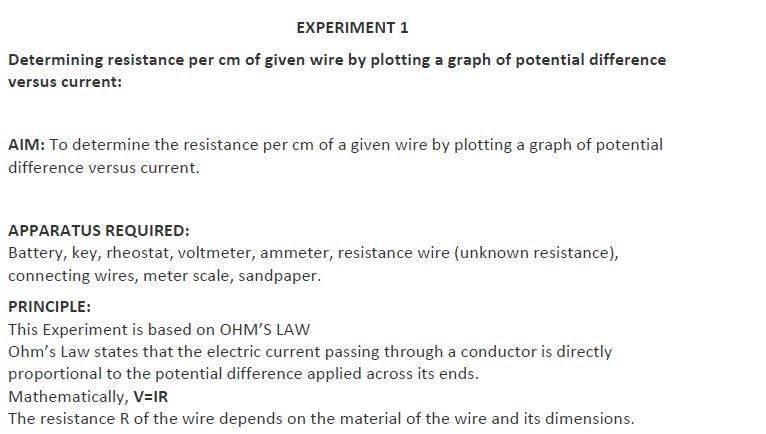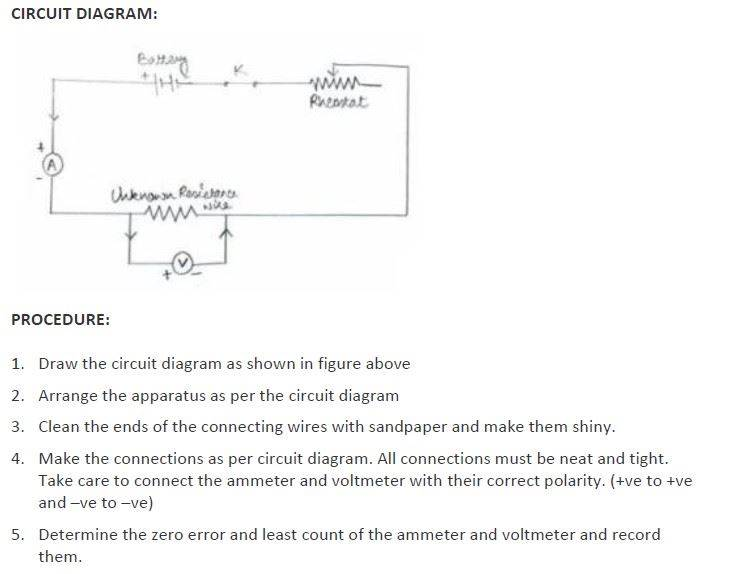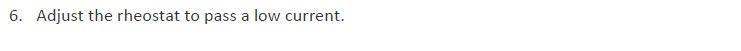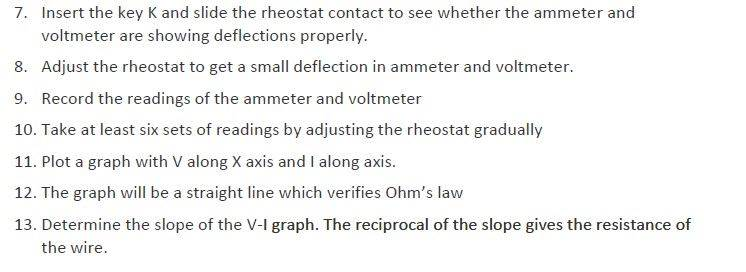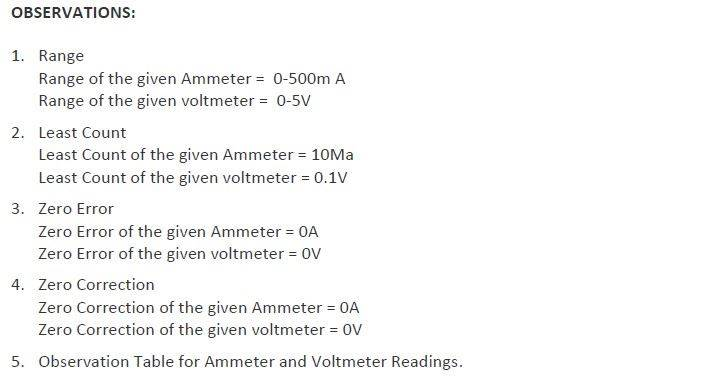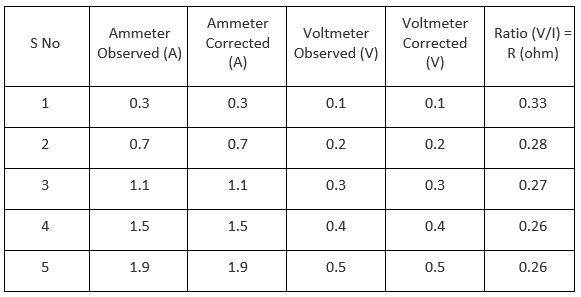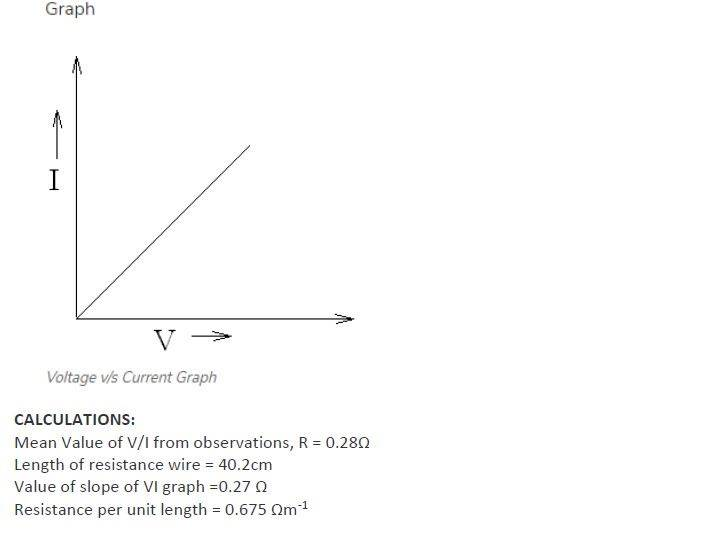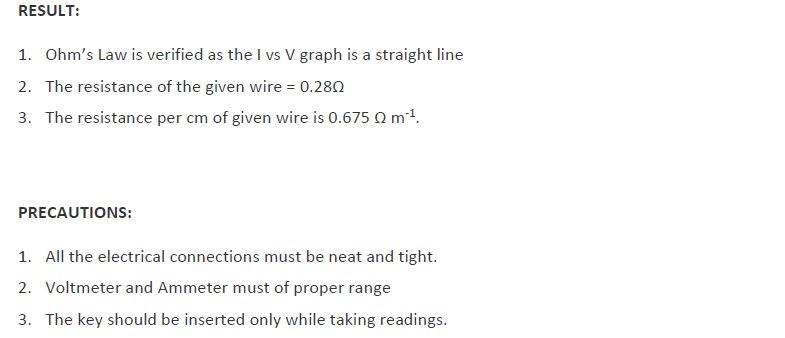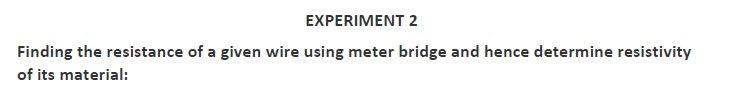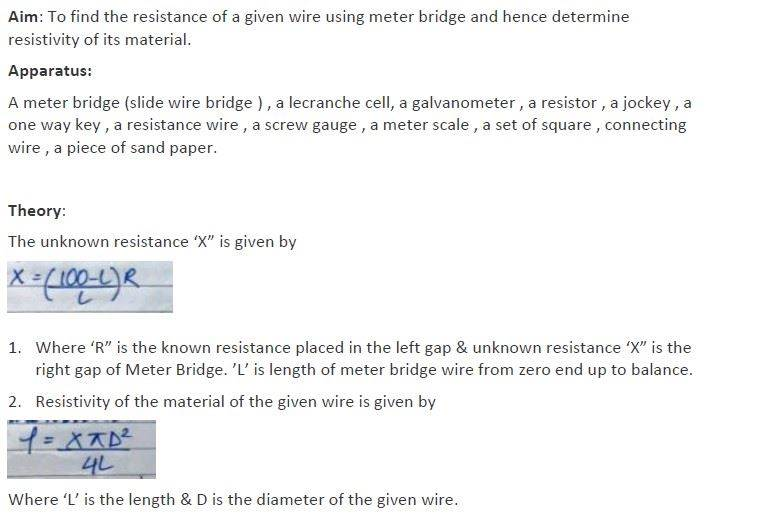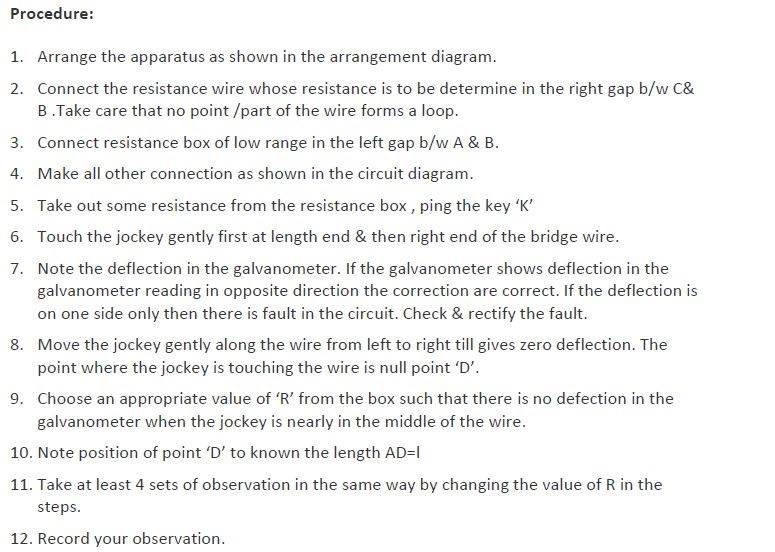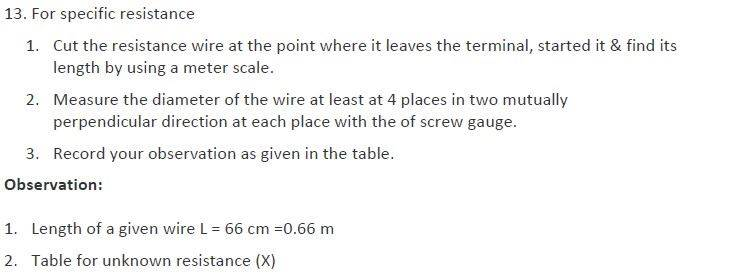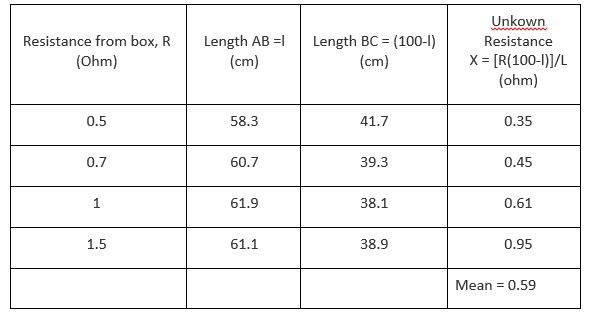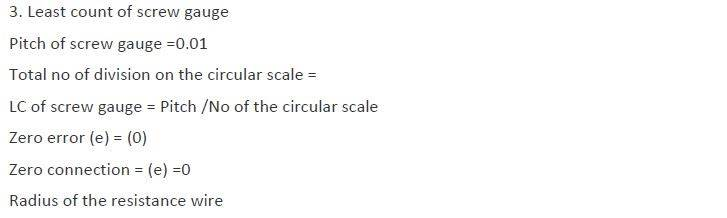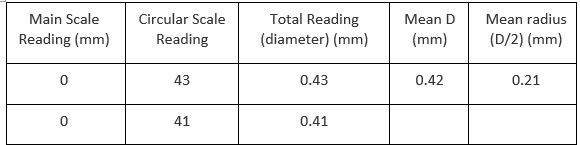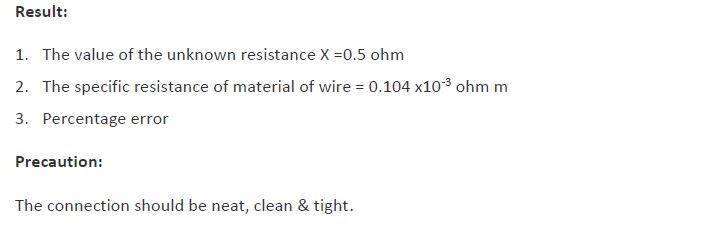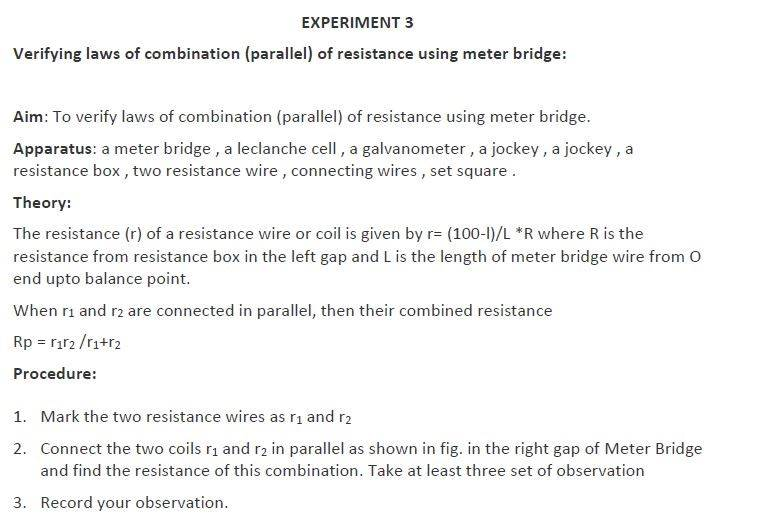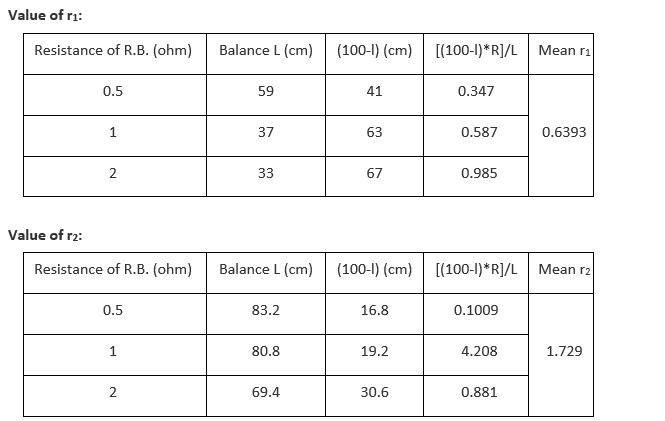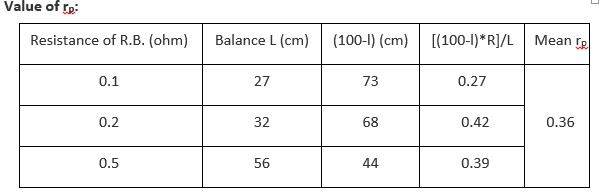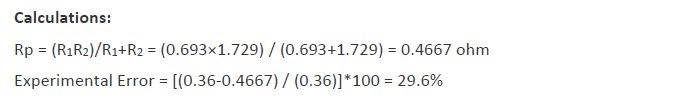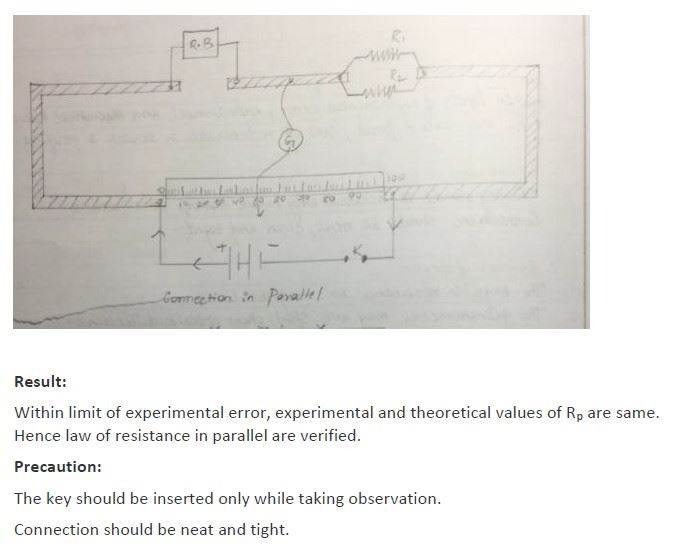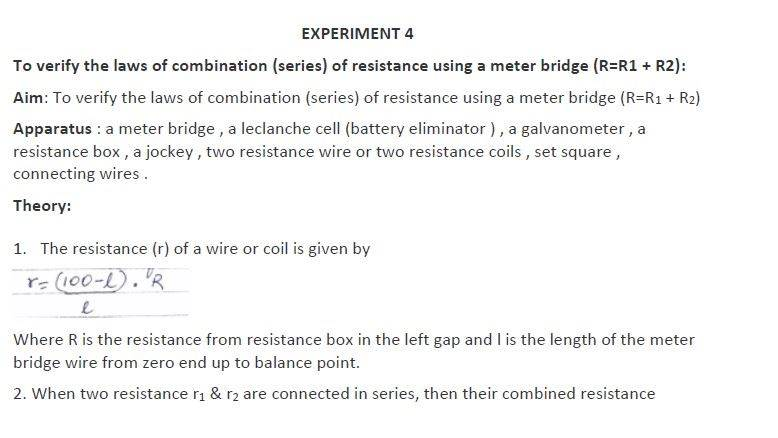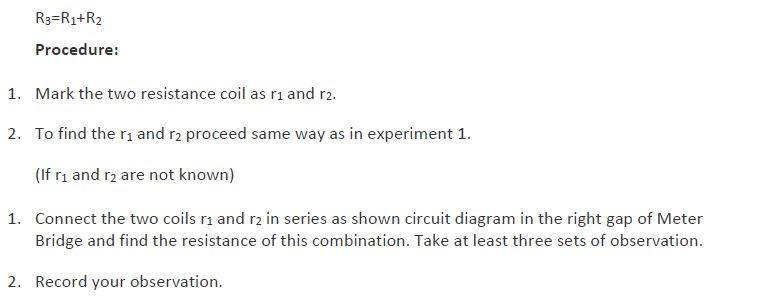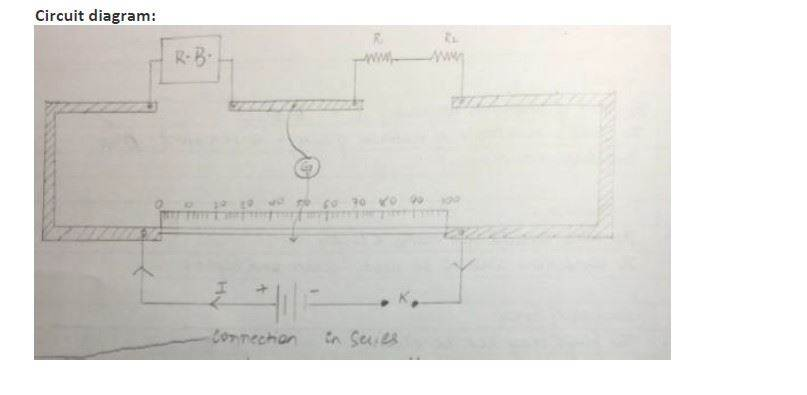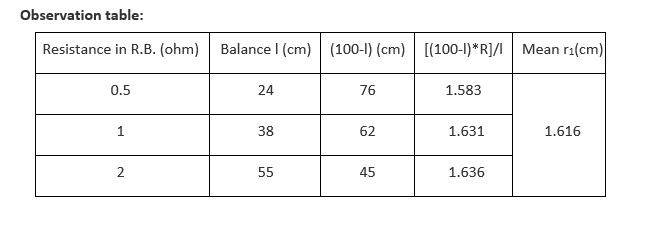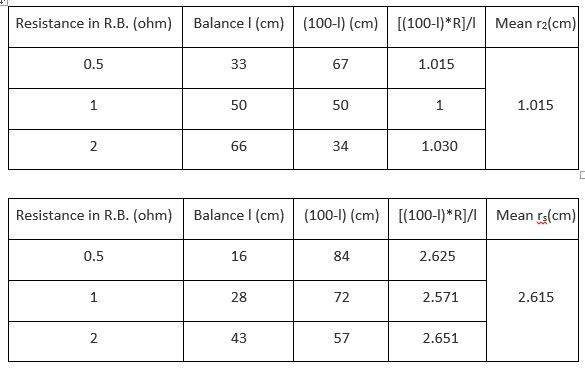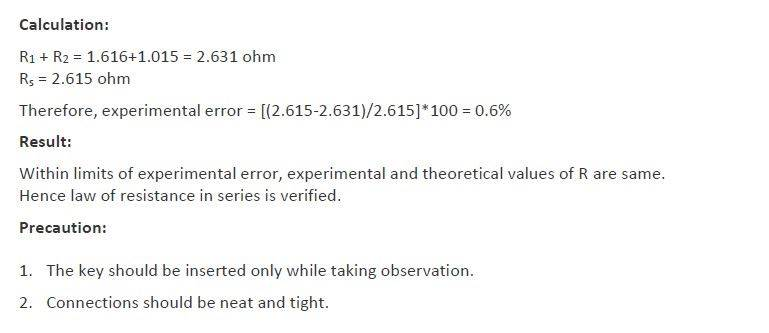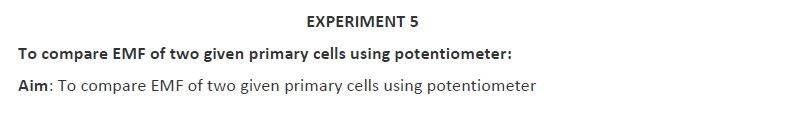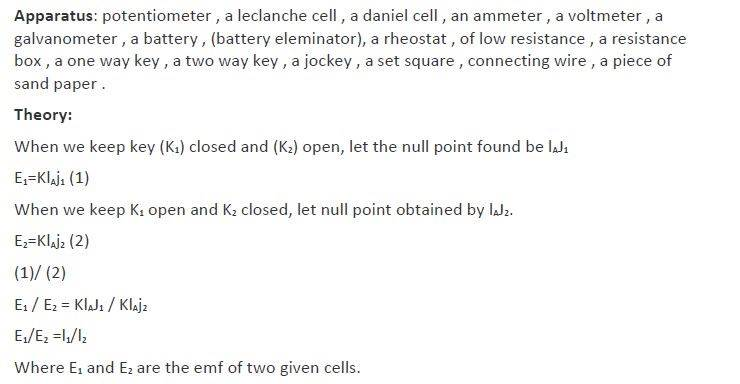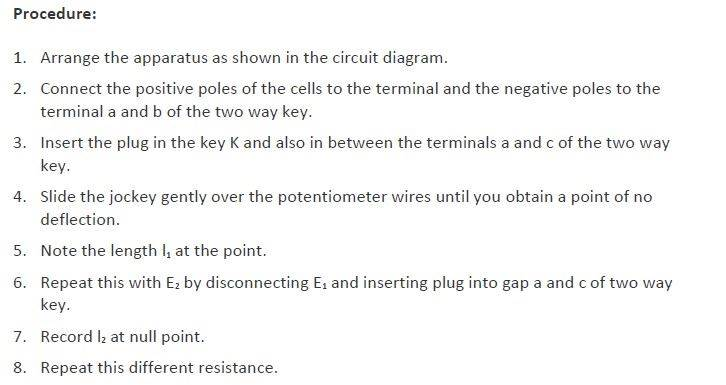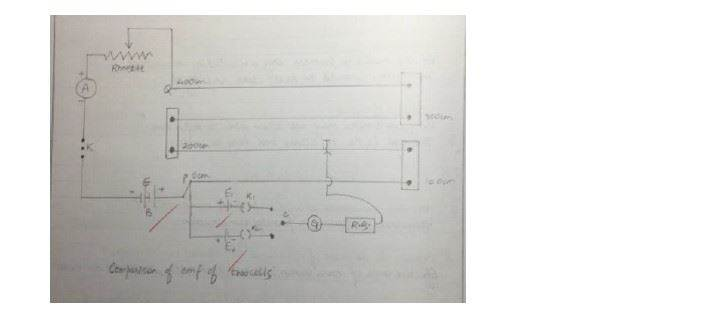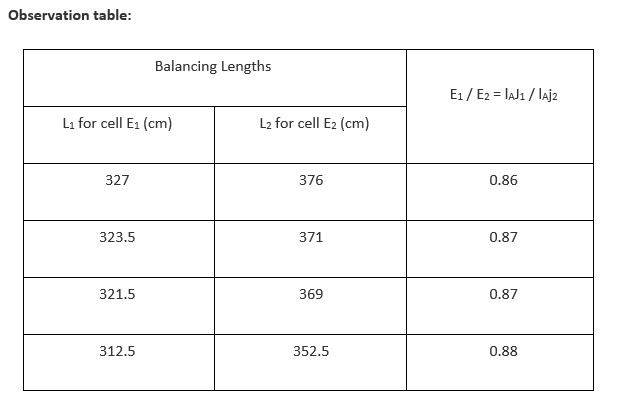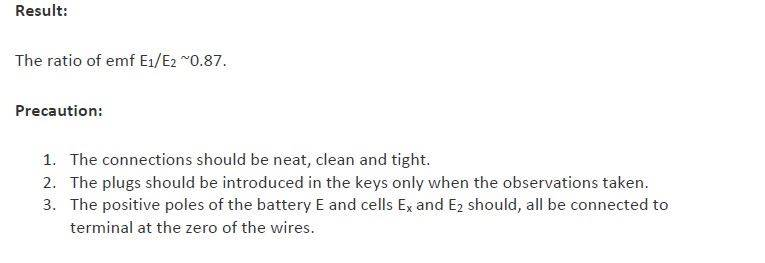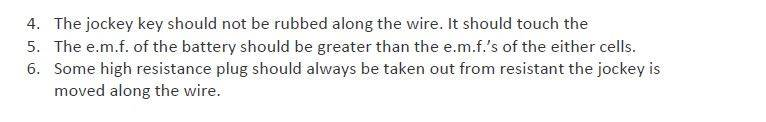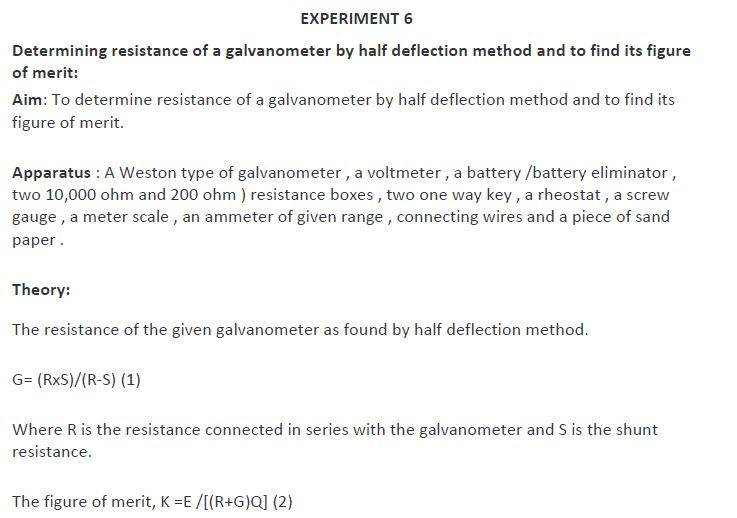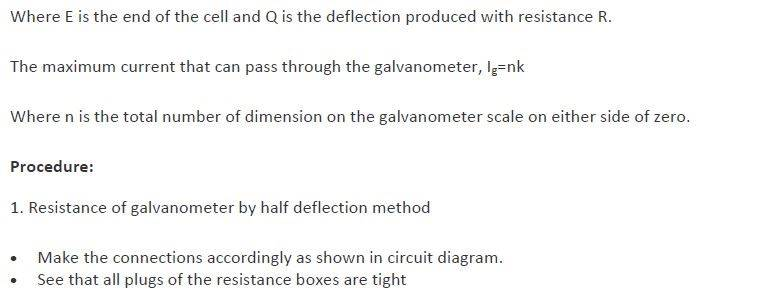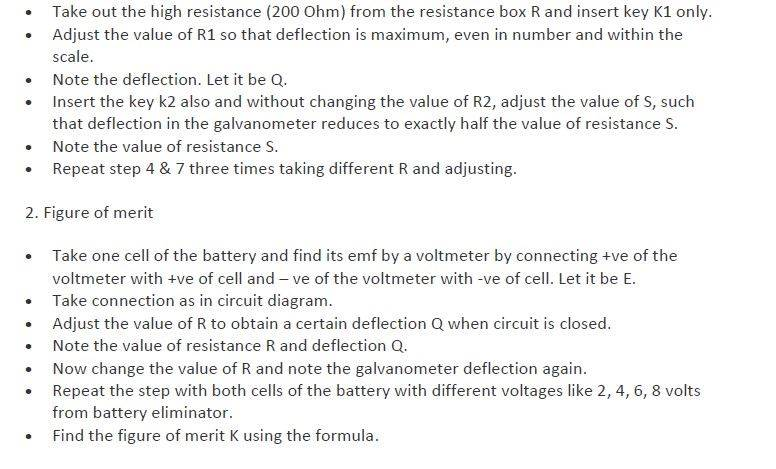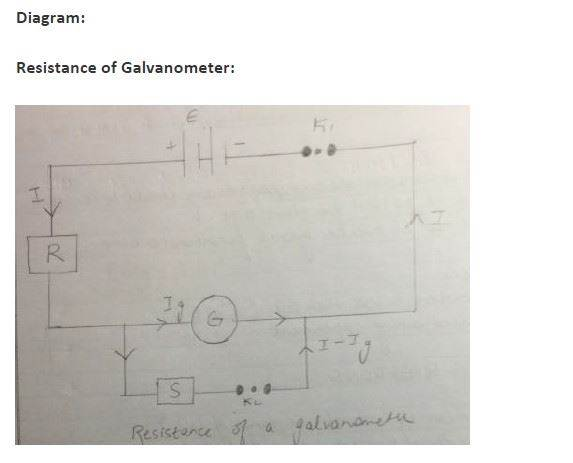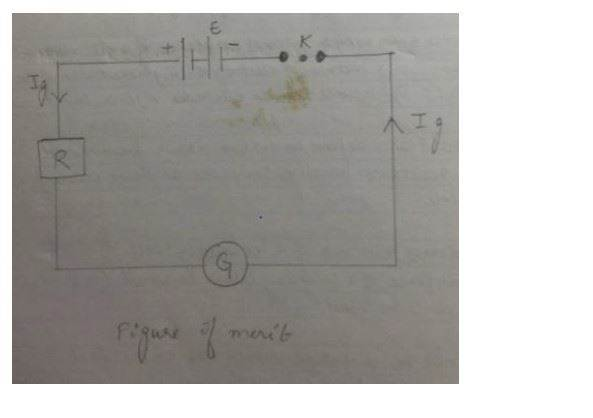Observation table :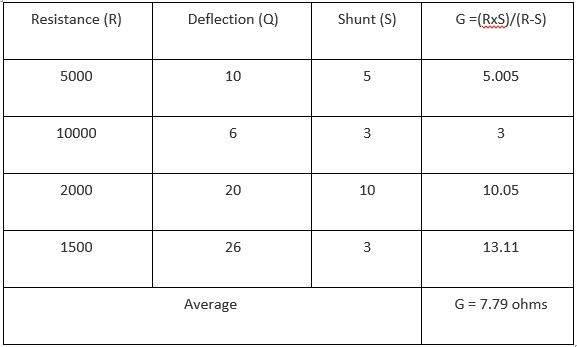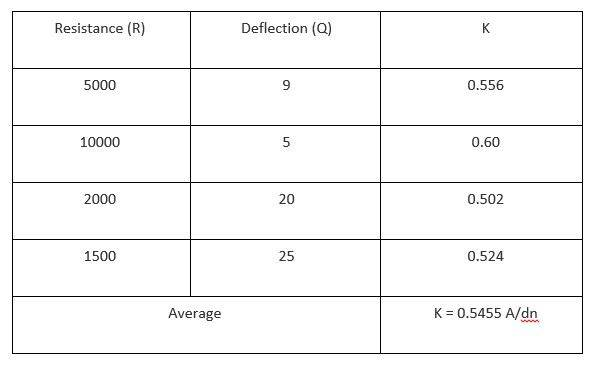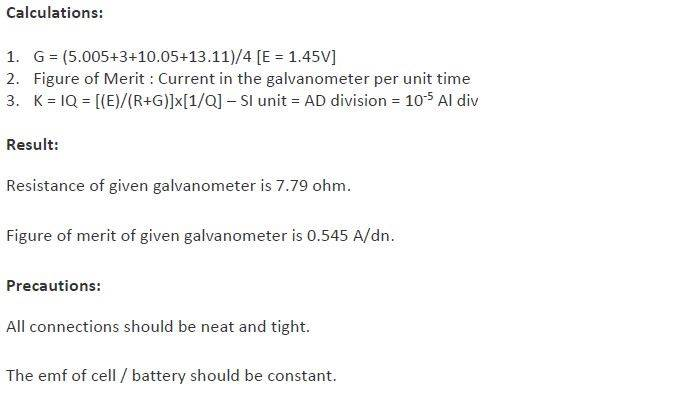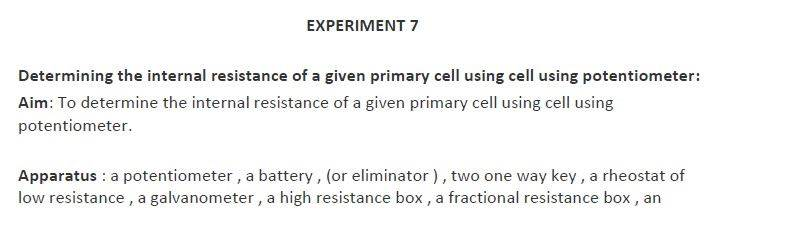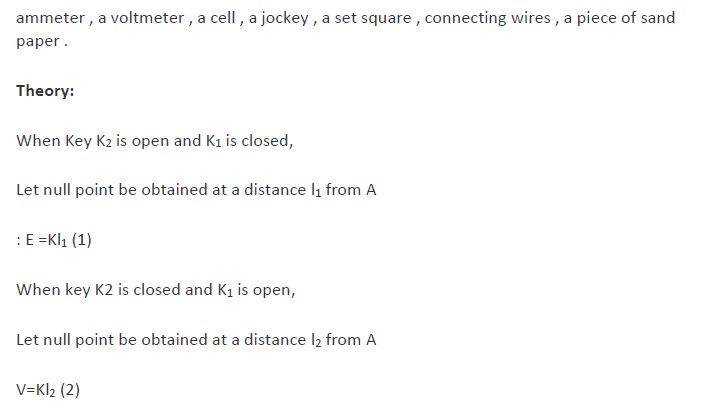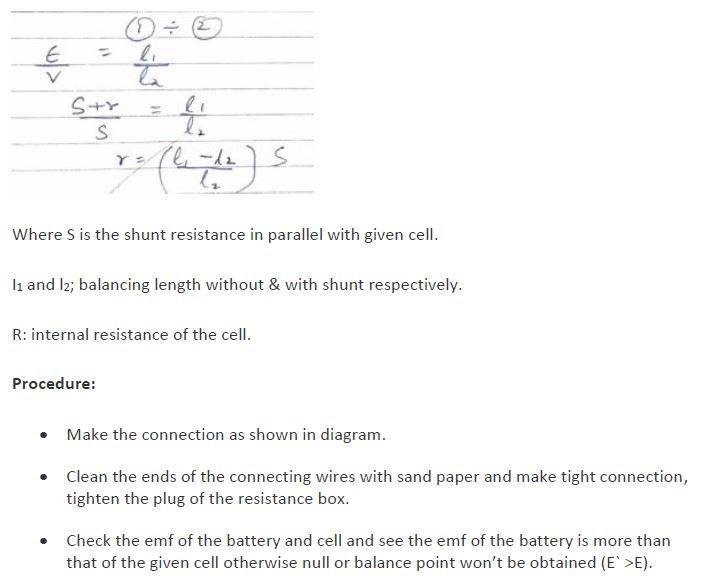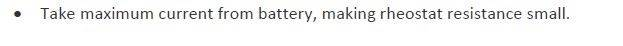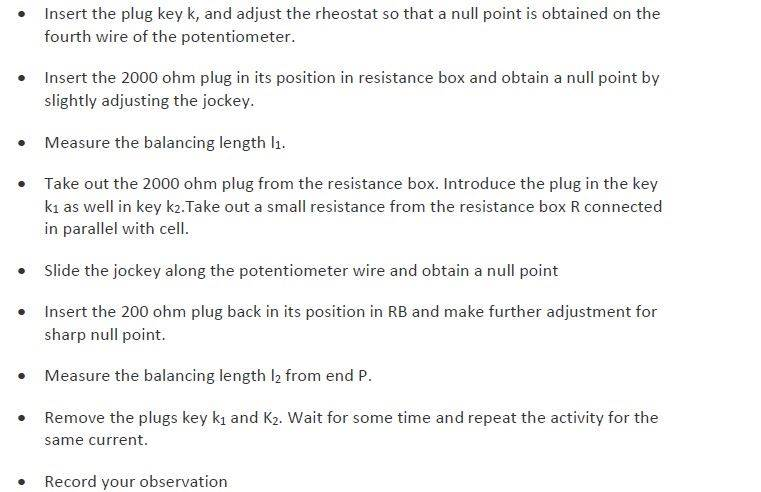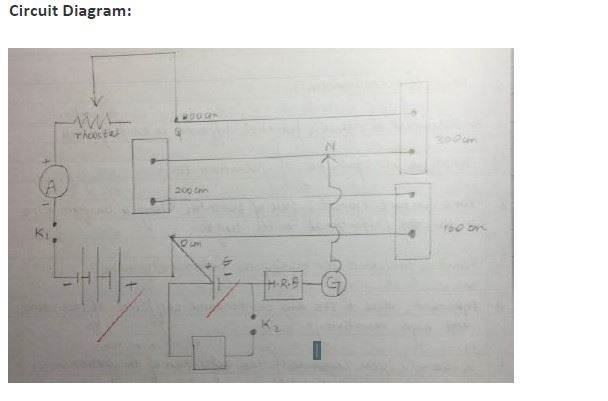Observation table :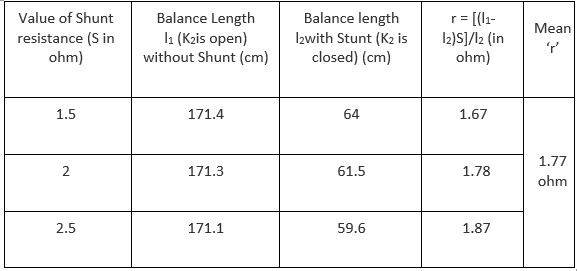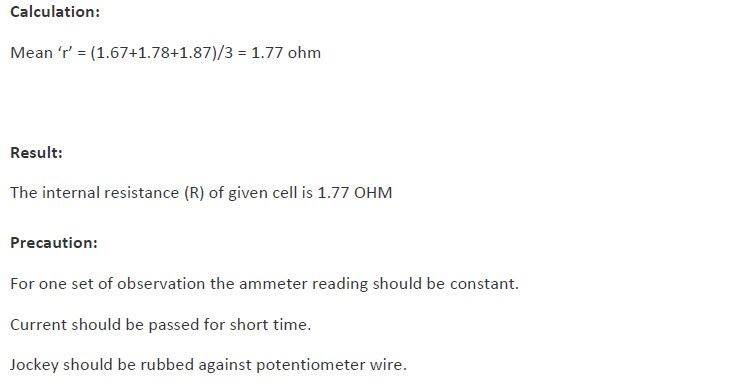Stay tuned to BYJU’S to get the latest notification on CBSE exams along with the CBSE syllabus, sample papers, marking scheme and more.